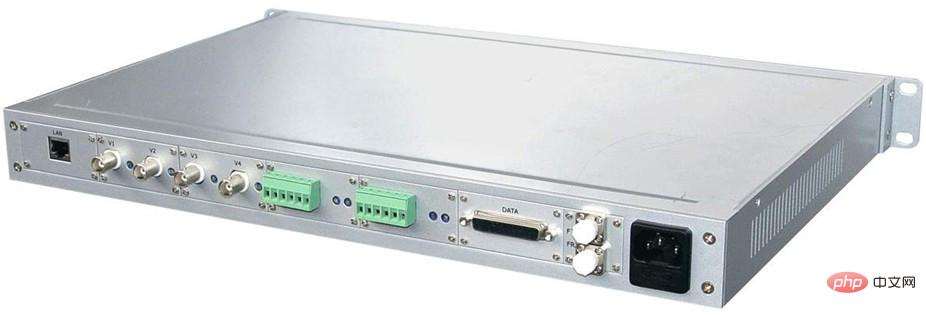# 1u机箱尺寸多少厘米

1u机箱尺寸为4.445厘米。u是一种表示组合式机架外部尺寸的单位，详细的尺寸由作为业界团体的美国电子工业协会（EIA）所决定，厚度以4.445cm为基本单位，1u就是4.445cm，2u则是1u得2倍为8.89cm，如此类推。1u机箱尺寸为4.445厘米。

u，unit的缩略语，是一种表示组合式机架外部尺寸的单位，详细尺寸由作为业界团体的美国电子工业协会（EIA）决定，厚度以4.445cm为基本单位，1u就是4.445cm，2u则是1u得2倍为8.89cm，如此类推。U是一种表示服务器外部尺寸的单位(计量单位：高度或厚度)，之所以要规定的尺寸是服务器的宽（48.26cm（19英寸））与高（1.75英寸（4.445cm）的倍数）。由于宽为19英寸，所以有时也将满足这一规定的机架称为“19英寸机架”。

1U=4.445*1=4.445厘米

2U=4.445*2=8.89厘米

3U=4.445*3=13.335厘米

4U=4.445*4=17.78厘米

5U=4.445*5=22.225厘米

6U=4.445*6=26.67厘米

8U=4.445*8=35.56厘米

12U=4.445*12=53.34厘米

16U=4.445*16=71.12厘米

20U=4.445*20=88.9厘米

24U=4.445*24=106.68厘米

28U=4.445*28=124.46厘米

32U=4.445*32=142.24厘米

36U=4.445*36=160.02厘米

40U=4.445*40=177.8厘米• 相关标签：厘米 机箱
• 相关文章

相关视频